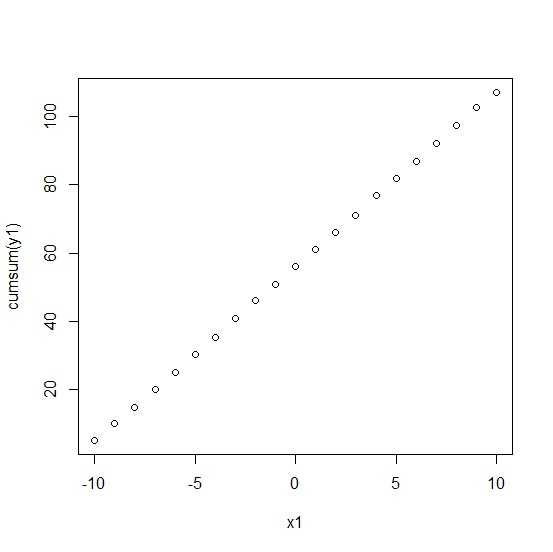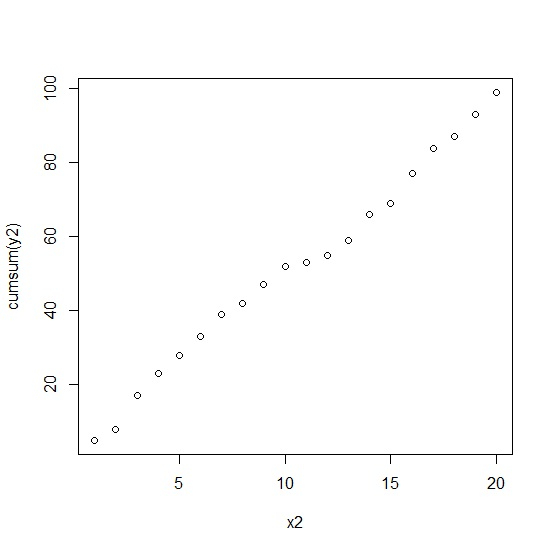# How to create a cumulative sum plot in base R?

To create a cumulative sum plot in base R, we can simply use plot function. For cumulative sums inside the plot, the cumsum function needs to be used for the variable that has to be summed up with cumulation. For example, if we have two vectors say x and y then the plot with cumulative sum plot can be created as plot(x,cumsum(y)).

## Example

x1<--10:10
y1<-rnorm(21,5,0.324)
plot(x1,cumsum(y1))

## Output## Example

x2<-1:20
y2<-rpois(20,5)
plot(x2,cumsum(y2))

## Output